HOME Electronics Directory Articles/ Tutorials eBooks About Us FORUM Links Contact Us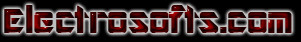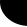Project Tutorial:                                                 Active Vibration Control using Fuzzy Logic

INTRODUCTION

I.      General introduction

An aircraft when airborne is subjected to aerodynamic forces on its structural components. These forces excite the resonances in the material of which the aircraft is made of and cause severe vibrations. It is important to study, analyze and measure the vibratory response of the aircraft structure. Further it is also important to control and suppress the vibration to tolerable levels to avoid catastrophic failure.

II.      Statement of the problem

Over the last decade, extensive research has been carried out,

·        To understand sources of vibration and characterize these source for their amplitude, frequency and statistical properties.

·        To develop electronic hardware like power sources, sensing and actuation amplifiers etc.

·        To acquire vibration signals from sensors to a computer using analog to digital converters and analyze them.

·        To actively suppress these vibrational signals using modern control concepts.

III.      Objectives of the study

Active Vibration Control (AVC) is a very demanding discipline. It consists of acquiring response signal from a vibrating structure using a sensor, Processing this signal and generating a control signal to an actuator attached on the structure in such a way as to suppress the vibrations. Its successful implementation requires a detailed knowledge of vibration engineering principle, fuzzy logic concepts, Computers, Control system design and electronics. The employment of active technology generally introduces some penalty like additional weight, increased maintenance costs, etc. these can be reduced with the development of

·        New kinds of integrated, multifunctional sensors and actuators working as components lying optimally in the load path (smart concept).

·        Though the controller can be either analog or digital, the most efficient form of controller is the digital controller, which makes use of digital techniques to compute the control signal.

·        The objective is to build the Fuzzy Rule based Active vibration Control in order process the available Vibration Data and generate a control signal based on the Fuzzy Rules.

IV.      Scope of the study

The scope of project is to develop software for signal acquisition and control law implementation using Fuzzy Rules. The major tasks involved in this are the following:

Ø      Source code is developed using ‘MATLAB FUZZY TOOLBOX’ to run off line codes for suppressing the vibration amplitudes.

Ø      Fuzzy Rules are implemented in ‘MATLAB’environment

V.      Methodology

Active Vibration Control (AVC) is the process of reducing existing levels of vibration by introduction of additional damping by means of one or more secondary or control actuators. The introduced vibration may achieve the required reduction by the way of introducing controlled vibration in antiphase to original levels.

In this project, fuzzy controller is used so as to suppress the vibrations. It is an off line controller that it makes use of the readings from vibration sensor stored in excel file format. These readings are vibration amplitude against frequency. These are the input to fuzzy controller which compares this with reference value and error is calculated. Each input is defined with 5 membership functions and passed through rule base generator to get output which is defuzzified to get the desired suppression in vibration amplitudes.

VI.      Organization of the thesis

This thesis introduces simple fuzzy logic controller designed for the vibration control using active vibration technique. Chapter 1 includes introduction to the vibrations, different types of vibration control techniques.

Chapter 2 gives fundamental fuzzy concepts and fuzzy inference system and its types. It also covers various steps involved in designing an inference system.

Chapter 3 includes simulating fuzzy controller using “FUZZY LOGIC TOOLBOX”. It also gives the detail description of the various functions used in designing this project.

Chapter 4 includes the block diagram of the active vibration control experiment and results obtained with various waveforms.

INTRODUCTION TO VIBRATIONS

Vibration

Vibrations are omnipresent in all mechanical systems. A vibration is a continuing change in the position of a body that follows slightly a regular pattern. It is the main characteristic that defines the motion in structures and machine components of any bulk structure. Force and stress in the vibrating bodies comprise the bulk of the vibrations. Vibrations are detrimental to system performance in many ways.

The study of source of vibration and their effects is necessary in the view of designer to develop structures that are free from vibration. Vibrations are to be isolated in systems because they lead to increased fatigue and degeneration of the system and the final result being the failure of the system. System performance becomes unpredictable in the wake of such of a plight.

Vibration in aerospace structures

Modern supersonic aircraft under different operating conditions are subjected to vibrations caused by the engine, aerodynamics turbulence, gust etc. this may excite some of the resonant modes of the structure to cause failure of the structure or to violated the efficiency and hence the system performance.

When an aircraft stationary on ground, there will be no vibrations experienced by it. Once the aircraft takes of vibrations are developed. As it takes of it experiences forces due to aerodynamic forces on its wings, fines, cockpit etc, which leads to undesirable effects. Some times these vibrations are so large that they lead to catastrophic failure that is, sudden failure of wings and fines of the aircraft. Thus it is imperative to control and reduce the vibration to the maxima possible extent

Effects of vibration and its control

The study of effect and control of vibration is necessary to develop structure that do not succumb to vibrations and fail under operating condition. The study also impresses the need to provide isolation of vibration for the safety of the personal and the equipment.

In order to study the behavior and effect of the structure the system under dynamic condition, curtain essential parameter are to be made note of. This could be done basically by two approaches namely,

·        analytical methods

·        vibration tests

Vibration tests are carried out to

·        Find response of the aircraft structure under various load condition and obtaining the characteristics of the structure in terms of frequency, damping and mode shapes.

·        Define the vibration environment.

·        Monitor and control a system.

Vibration could be eliminated to certain extent using the following methods.

·        Removal of the external excitations.

·        Use of shock absorbers.

·        Use of dynamic absorbers.

·        Providing proper vibration isolation.

Vibration method could be classified as.

·        Passive vibration control

·        Active vibration control

Passive vibration control

The physical parameters after structure such as it’s mass, damping coefficient and stiffness of the structure determined the response of the system to vibrations. Changing this parameters can be considered as a redesigning process for an already existing structure to produce more desirable response this is passive vibration control method.

The frequency of such a system is derived from the equation 1,

Frequency=1/2∏×√(k/m)cps……………(1)

Where m-mass

K-stiffness

The constraints mass (m), damping coefficient(c) and the stiffness (k) are such that only two of them can be varied because damping co efficient for given structure is constant.

The response of the structure will be altered far from desired response because of many reasons; one of them being only a 10 % vibration is possible in the desired value of the mass.

When the frequency of vibration is equal to natural frequency of the passive system, resonance occurs. This leads to failure of the flexible structures such as satellite truss or a peace of vibrating machinery.

They can be bulky and heavy when used at low frequencies (bellow 500 Hz) since; size and mass of passive method usually depend on acoustic wavelength.

Active vibration control

Active vibration control aims at minimizing the response of vibration rather than to control the source of vibration. It is a related technique that resembles active noise control. They use actuators, which are electrochemical devices that control the response of the elastic medium. Actuators are the secondary vibration source (shakers, piezoceramic patches etc), which can modify the vibration. Actuators used in active vibration control can be broadly classified as,

1)      Fully active

2)      Semi active

1) Fully active actuators supply mechanical power to the system. They can be used to generate a secondary vibrational response in the linear mechanical system. This reduces the overall response by destructive interference with the original response of the system, caused by the primary source of vibration.

2) Semi active actuators behave essentially as passive systems. Their use in active control systems steam from the fact that their passive mechanical properties can be adjusted by the application of a control signal and in such systems, one of the special types active control is adaptive control system.

Active vibration control is brought about using the “Smart Structure Technology”. Generally the methodology involved in vibration reduction is achieved by introducing additional vibrations in anti-phase to the structure through a controller-actuator combination.

Active vibration Control is a process of reducing existing levels of vibration by means of one or more secondary control sources.

Summary

In this chapter we studied vibration as a continuing change in the position of the body that follows slightly regular pattern.  Causes for the vibrations in aerospace structures and different applications where control of vibration is of prime importance. Also this chapter covered the effects of vibrations and various controlling techniques.

In this project we are implementing ‘active vibration control’ (AVC) concept using fuzzy logic. So its very important to know the basic concepts of fuzzy logic. All these are covered in detail in the next chapter.

ACTIVE CONTROL EXPERIMENT

In the present study, it is the off-line vibration control which is implemented in MATLAB environment using fuzzy toolbox. The block diagram is shown below in figure 29: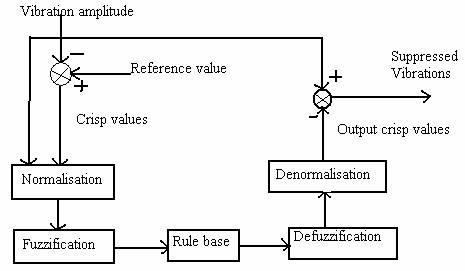Figure : Block diagram of vibration control using fuzzy logic.

The vibrations are read for various frequencies and compared with the reference value to generate error signal. Now these two vibration and error signals both are normalized then for each of the input 5 fuzzy rules are written and are executed using FIS file. 25 rules are written in rule editor and for output also 5 membership functions are defined.  Now these output values are renormalized to get the crisp values which are combined with vibration amplitudes which suppress them to reference value.

.

FLOWCHART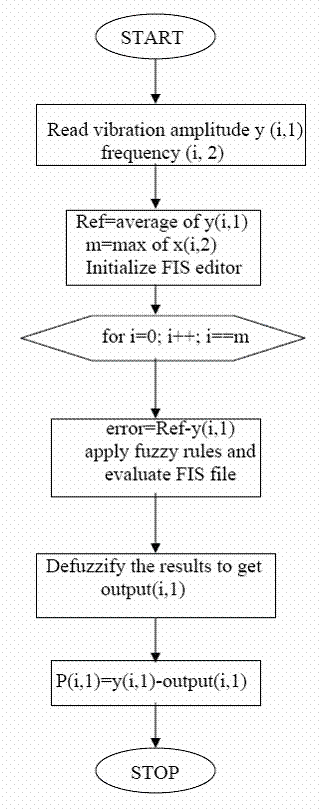RESULTS

The frequency domain signals captured from vibration sensor and are stored in excel file form. Further the acquired data is processed to suppress the vibration amplitudes to the reference values.

As the present work is based on amplitude (displacement) control concept, the efficiency of the fuzzy controller is assessed with respect to achieved reduction in amplitude. The vibration amplitude is reduced to reference level very significantly and the input and out plots are shown below.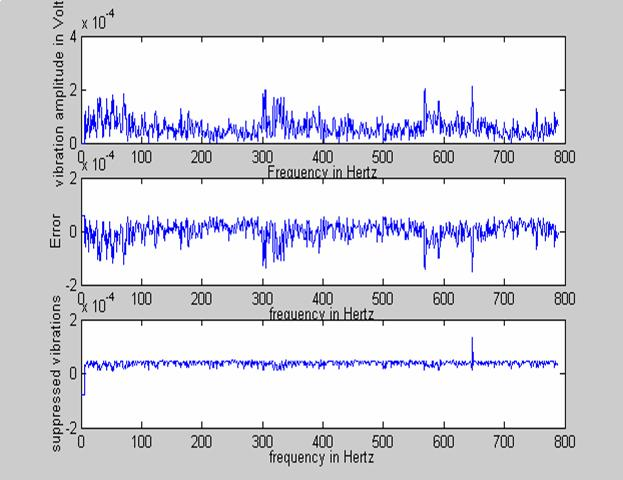Figure Input vibrations, error and output suppressed vibrations.

CONCLUSION

The present study has helped to understand the active control concept that can be employed for the vibration control. The vibrations of the systems are much effectively suppressed using fuzzy logic controller algorithm in MATLAB. The experimental results are shown in figure 31.

Since fuzzy does not make use of the mathematical model it is much easier in controlling non-linear systems. For variety of applications like aircraft/aerospace and robotics etc. now a days it is required economical and practical solution of vibration control. Keeping this in mind our project is built using fuzzy logic in MATLAB environment which makes cost effective.

As the fuzzy controller is easy to implement in real time, fuzzy rules can be used to design a controller and implemented as a control for structural vibration suppression purposes.

Like everything in this world, this product also has scope of improvements. It is used for off line control and only two parameters are taken into consideration i.e. amplitude and frequency. But it is possible to develop for real time application. Also we can consider more parameters such as phase. The future work can be attempted to develop an Active Vibration Control (AVC) scheme for real time applications like flexible robotic arm.

Also it can be attempted develop AVC with Multi Input Multi Output (MIMO) concept for aircraft structural vibration control application.

(You can contact author through email: vadiraj@electrosofts.com )Web electroSofts.com